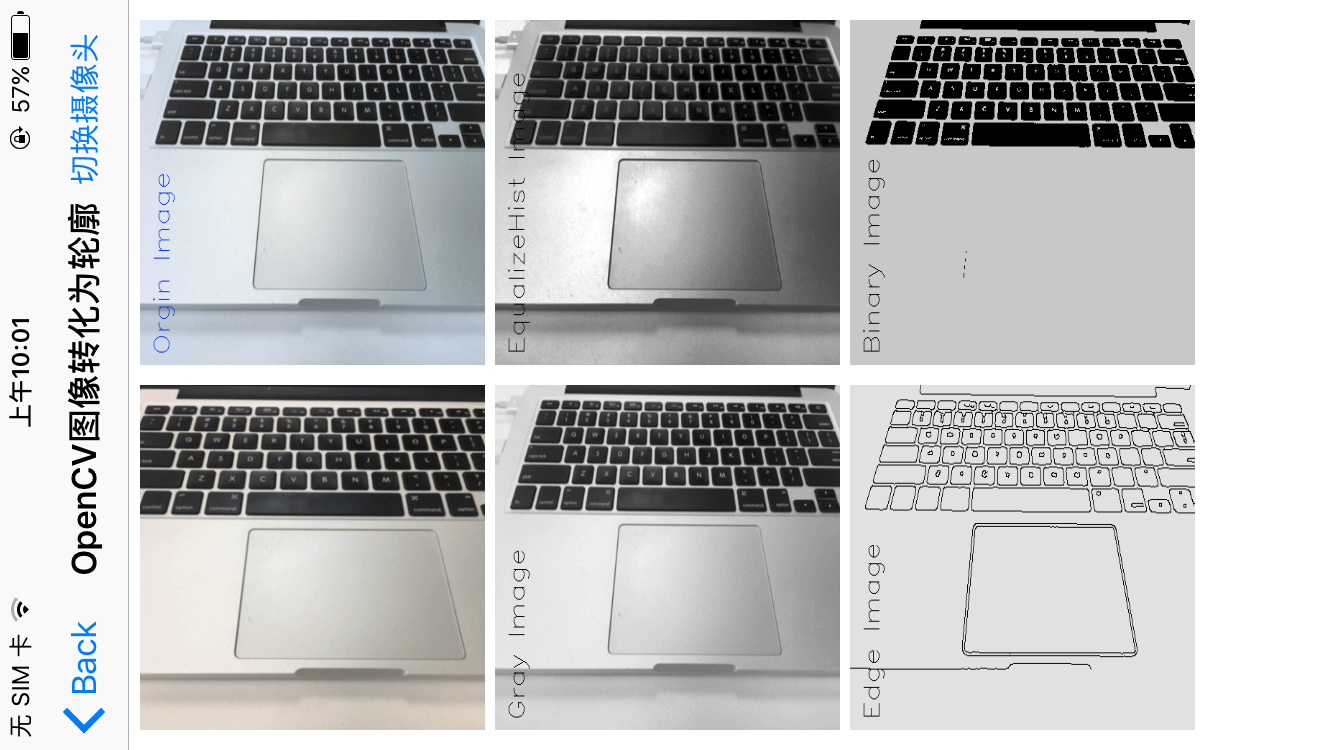## iOS通过摄像头动态识别图像

### 前言：

• 基于规则运算的图像识别，例如颜色形状等模板匹配方法
• 基于统计的图像识别。例如机器学习ML，神经网络等人工智能方法

### 实现效果图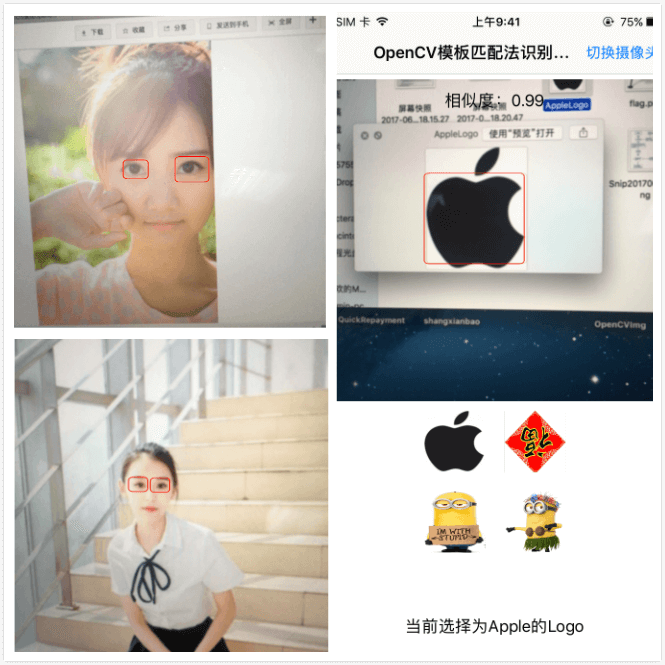### 一、方案选择

#### 1.1、iOS客户端快速实现图像识别的两种方案:• AlphaGo战胜世界围棋冠军，人工智能大火，谷歌去年开源了其用来制作AlphaGo的深度学习系统Tensorflow，而且Tensorflow支持了iOS，Android等移动端。
• OpenCV于1999年由Intel建立的，跨平台的开源计算机视觉库，主要由C和C++代码构成，有Python、Ruby、MATLAB等语言的接口，支持iOS，Android等移动设备。
• 显而易见，虽然都是开源库，都支持机器学习ML，但从推出时间，代码迭代，资料的丰富度，以及前辈已经给踩平的坑来讲，OpenCV是成熟的，应该首先选择的。

#### 1.2、OpenCV中实现图像识别的方法对比:

• 模板匹配
• 适合固定的场景、物体或特定形状的图片识别
• eg1:某公司的Logo图标，假设图标是不变的，也适用
• eg2:适用于某个图片是另外一张大图的一部分的场景
• eg3:例如五角星形状固定，可转换为边框匹配
• 特征点检测
• 适合标记两幅图片中相同的特征点
• eg1:有相同部分的照片拼接，视频运动追踪
• eg2:例如全景图片的拼接，长图的拼接
• eg3:监控视频中的目标跟踪
• 基于机器学习ML的训练分类器用来分类的方法
• 此方法依赖于训练数据，给机器提供大量包含目标的正确数据和不包含目标的错误背景数据，让机器来总结提取特征，适合识别某类有多种状态的场景或物体识别
• eg1:人脸识别、人眼识别，身体识别等等
• eg2:支付宝扫福，福字有成千上万种写法

### 二、OpenCV集成

#### 2.1、iOS项目集成OpenCV，主要有两种方法

• 方法1: 从OpenCV官网下载opencv2.framework框架，然后拖入即可，导入依赖的库，具体集成方法见我的另外一篇文章iOS集成OpenCV
• 方法2: CocoaPods方式集成，Pod文件中配置pod ‘OpenCV’，而实验证明，用CocoaPods方式配置虽然简单，但自动配置的不正确，存在名称重复等大量的问题。

### 三、模板匹配法

#### 3.1、获取视频图像

``````#pragma mark - 获取视频帧，处理视频
- (void)captureOutput:(AVCaptureOutput *)captureOutput didOutputSampleBuffer:(CMSampleBufferRef)sampleBuffer fromConnection:(AVCaptureConnection *)connection;
``````

#### 3.2、将视频帧转换为cv::Mat矩阵

``````#pragma mark - 将CMSampleBufferRef转为cv::Mat
+(cv::Mat)bufferToMat:(CMSampleBufferRef) sampleBuffer{
CVImageBufferRef imgBuf = CMSampleBufferGetImageBuffer(sampleBuffer);

//锁定内存
CVPixelBufferLockBaseAddress(imgBuf, 0);
// get the address to the image data
void *imgBufAddr = CVPixelBufferGetBaseAddress(imgBuf);

// get image properties
int w = (int)CVPixelBufferGetWidth(imgBuf);
int h = (int)CVPixelBufferGetHeight(imgBuf);

// create the cv mat
cv::Mat mat(h, w, CV_8UC4, imgBufAddr, 0);

//转换为灰度图像
cv::Mat edges;
cv::cvtColor(mat, edges, CV_BGR2GRAY);

//旋转90度
cv::Mat transMat;
cv::transpose(mat, transMat);

//翻转,1是x方向，0是y方向，-1位Both
cv::Mat flipMat;
cv::flip(transMat, flipMat, 1);

CVPixelBufferUnlockBaseAddress(imgBuf, 0);

return flipMat;
}
``````

#### 3.3、视频帧矩阵转换为灰度矩阵

``````//将图片转换为灰度的矩阵
-(cv::Mat)initTemplateImage:(NSString *)imgName{
UIImage *templateImage = [UIImage imageNamed:imgName];
cv::Mat tempMat;
UIImageToMat(templateImage, tempMat);
//cv::cvtColor(tempMat, tempMat, CV_BGR2GRAY);
return tempMat;
}
``````

#### 3.4、视频帧矩阵与模板矩阵对比

``````/**
对比两个图像是否有相同区域

@return 有为Yes
*/
-(BOOL)compareInput:(cv::Mat) inputMat templateMat:(cv::Mat)tmpMat{
int result_rows = inputMat.rows - tmpMat.rows + 1;
int result_cols = inputMat.cols - tmpMat.cols + 1;

cv::Mat resultMat = cv::Mat(result_cols,result_rows,CV_32FC1);
cv::matchTemplate(inputMat, tmpMat, resultMat, cv::TM_CCOEFF_NORMED);

double minVal, maxVal;
cv::Point minLoc, maxLoc, matchLoc;
cv::minMaxLoc( resultMat, &minVal, &maxVal, &minLoc, &maxLoc, cv::Mat());
//    matchLoc = maxLoc;
//    NSLog(@"min==%f,max==%f",minVal,maxVal);
dispatch_async(dispatch_get_main_queue(), ^{
self.similarLevelLabel.text = [NSString stringWithFormat:@"相似度：%.2f",maxVal];
});

if (maxVal > 0.7) {
//有相似位置，返回相似位置的第一个点
currentLoc = maxLoc;
return YES;
}else{
return NO;
}
}
``````

#### 3.5、模板匹配法优化

``````//图像金字塔分级放大缩小匹配，最大0.8*相机图像，最小0.3*tep图像
-(NSArray *)compareByLevel:(int)level CameraInput:(cv::Mat) inputMat{
//相机输入尺寸
int inputRows = inputMat.rows;
int inputCols = inputMat.cols;

//模板的原始尺寸
int tRows = self.templateMat.rows;
int tCols = self.templateMat.cols;

NSMutableArray *marr = [NSMutableArray array];

for (int i = 0; i < level; i++) {
//取循环次数中间值
int mid = level*0.5;
//目标尺寸
cv::Size dstSize;
if (i<mid) {
//如果是前半个循环，先缩小处理
dstSize = cv::Size(tCols*(1-i*0.2),tRows*(1-i*0.2));
}else{
//然后再放大处理比较
int upCols = tCols*(1+i*0.2);
int upRows = tRows*(1+i*0.2);
//如果超限会崩，则做判断处理
if (upCols>=inputCols || upRows>=inputRows) {
upCols = tCols;
upRows = tRows;
}
dstSize = cv::Size(upCols,upRows);
}
//重置尺寸后的tmp图像
cv::Mat resizeMat;
cv::resize(self.templateMat, resizeMat, dstSize);
//然后比较是否相同
BOOL cmpBool = [self compareInput:inputMat templateMat:resizeMat];

if (cmpBool) {
NSLog(@"匹配缩放级别level==%d",i);
CGRect rectF = CGRectMake(currentLoc.x, currentLoc.y, dstSize.width, dstSize.height);
NSValue *rValue = [NSValue valueWithCGRect:rectF];
[marr addObject:rValue];
break;
}
}
return marr;
}
``````

#### 3.5、模板匹配法实现效果图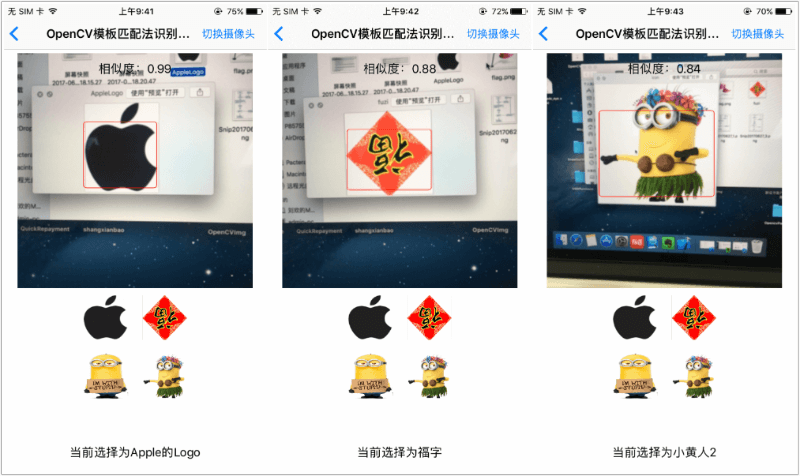### 四、机器学习ML训练分类器方法识别图像

#### 4.2、加载训练好的分类器

``````cv::CascadeClassifier icon_cascade;//分类器
``````

``````    //加载训练文件
NSString *bundlePath = [[NSBundle mainBundle] pathForResource:@"haarcascade_eye_tree_eyeglasses.xml" ofType:nil];
cv::String fileName = [bundlePath cStringUsingEncoding:NSUTF8StringEncoding];

BOOL isSuccessLoadFile = icon_cascade.load(fileName);
isSuccessLoadXml = isSuccessLoadFile;
if (isSuccessLoadFile) {
NSLog(@"Load success.......");
}else{
NSLog(@"Load failed......");
}
``````

#### 4.3、使用分类器识别图像

``````//获取计算出的标记的位置，保存在数组中
-(NSArray *)getTagRectInLayer:(cv::Mat) inputMat{
if (inputMat.empty()) {
return nil;
}
//图像均衡化
cv::equalizeHist(inputMat, inputMat);
//定义向量，存储识别出的位置
std::vector<cv::Rect> glassess;
//分类器识别
icon_cascade.detectMultiScale(inputMat, glassess, 1.1, 3, 0);
//转换为Frame，保存在数组中
NSMutableArray *marr = [NSMutableArray arrayWithCapacity:glassess.size()];
for (NSInteger i = 0; i < glassess.size(); i++) {
CGRect rect = CGRectMake(glassess[i].x, glassess[i].y, glassess[i].width,glassess[i].height);
NSValue *value = [NSValue valueWithCGRect:rect];
[marr addObject:value];
}
return marr.copy;
}
``````

#### 4.4、分类器识别图像效果图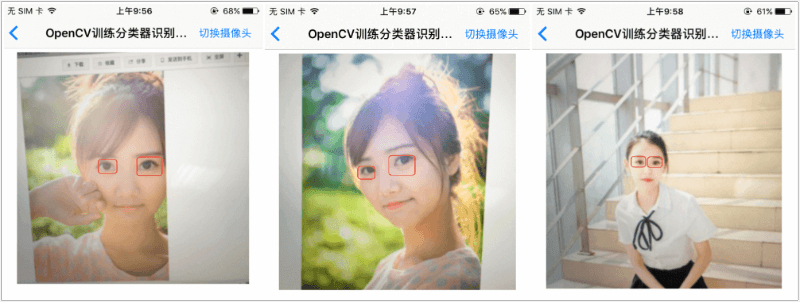### 五、Demo中的图像处理

#### 5.1、使用系统CIFilter高斯模糊视频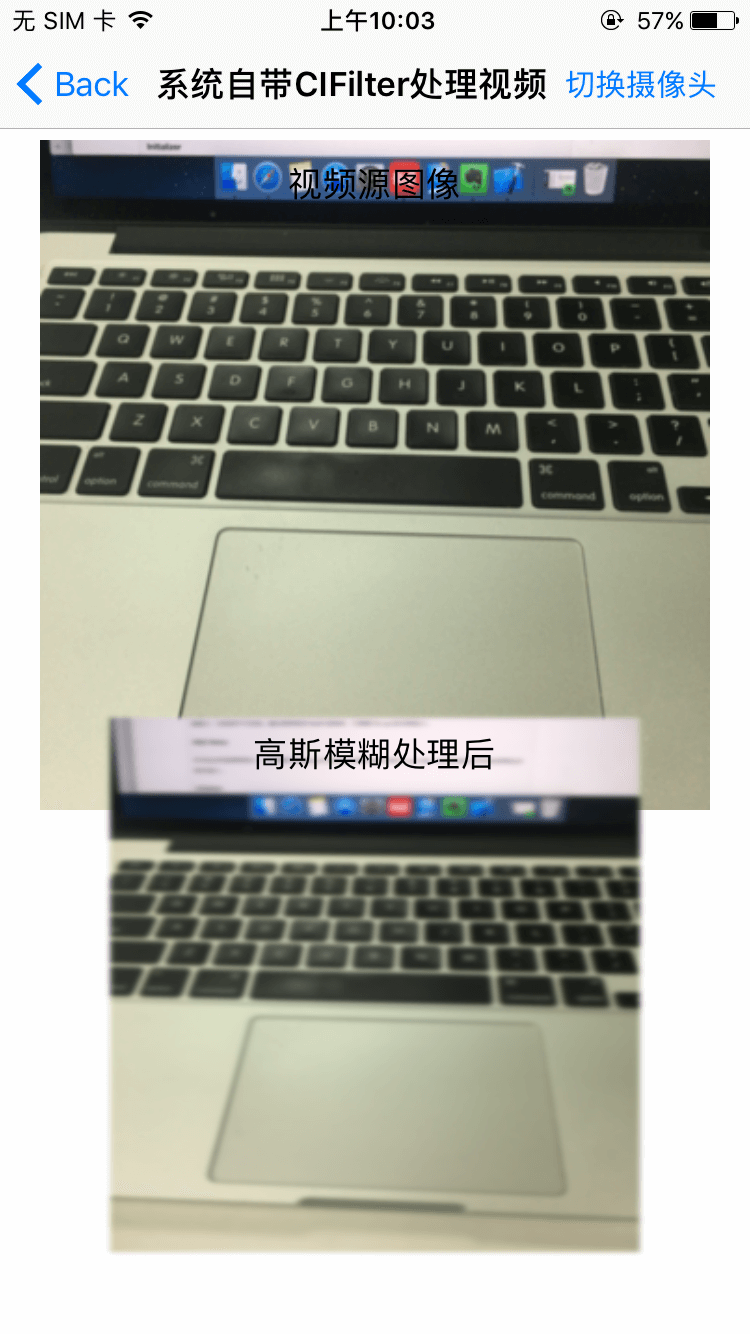#### 5.2、使用OpenCV处理视频图像

• 原图像
• 直方图均衡化
• 图像二值化
• 摄像头预览
• 灰度图
• 轮廓图